step

Step response plot of dynamic system; step response data

Description

Step Response Plots

example

step(sys) plots the response of a dynamic system model to a step input of unit amplitude. The model sys can be continuous- or discrete-time, and SISO or MIMO. For MIMO systems, the plot displays the step responses for each I/O channel. step automatically determines the time steps and duration of the simulation based on the system dynamics.

example

step(sys,tFinal) simulates the step response from t = 0 to the final time t = tFinal. The function uses system dynamics to determine the intervening time steps.

example

step(sys,t) plots the step response at the times that you specify in the vector t.

example

step(sys1,sys2,...,sysN,___) plots the step response of multiple dynamic systems on the same plot. All systems must have the same number of inputs and outputs. You can use multiple dynamic systems with any of the previous input-argument combinations.

example

step(sys1,LineSpec1,...,sysN,LineSpecN,___) specifies a color, line style, and marker for each system in the plot. You can use LineSpec with any of the previous input-argument combinations. When you need additional plot customization options, use stepplot instead.

example

step(___,opts) specifies additional options for computing the step response, such as the step amplitude or input offset. Use stepDataOptions to create the option set opts. You can use opts with any of the previous input-argument and output-argument combinations.

Step Response Data

example

y = step(sys,t) returns the step response of a dynamic system model sys at the times specified in the vector t. This syntax does not draw a plot.

[y,tOut] = step(sys) also returns a vector of times tOut corresponding to the responses in y. If you do not provide an input vector t of times, step chooses the length and time step of tOut based on the system dynamics.

[y,tOut] = step(sys,tFinal) computes the step response up to the end time tFinal. step chooses the time step of tOut based on the system dynamics.

[y,t,x] = step(sys) also returns the state trajectories x, when sys is a state-space model such as an ss or idss model.

example

[y,t,x,ysd] = step(sys) also computes the standard deviation ysd of the step response y, when sys is an identified model such as an idss, idtf, or idnlarx model.

example

[___] = step(___,opts) specifies additional options for computing the step response, such as the step amplitude or input offset. Use stepDataOptions to create the option set opts. You can use opts with any of the previous input-argument and output-argument combinations.

Examples

collapse all

Plot the step response of a continuous-time system represented by the following transfer function.

$\mathrm{sys}\left(\mathit{s}\right)=\frac{4}{{\mathit{s}}^{2}+2\mathit{s}+10}.$

For this example, create a tf model that represents the transfer function. You can similarly plot the step response of other dynamic system model types, such as zero-pole gain (zpk) or state-space (ss) models.

sys = tf(4,[1 2 10]);

Plot the step response.

step(sys)The step plot automatically includes a dotted horizontal line indicating the steady-state response. In a MATLAB® figure window, you can right-click on the plot to view other step-response characteristics such as peak response and settling time. For more information about these characteristics, see stepinfo (Control System Toolbox).

Plot the step response of a discrete-time system. The system has a sample time of 0.2 s and is represented by the following state-space matrices.

A = [1.6 -0.7;
1  0];
B = [0.5; 0];
C = [0.1 0.1];
D = 0;

Create the state-space model and plot its step response.

sys = ss(A,B,C,D,0.2);
step(sys)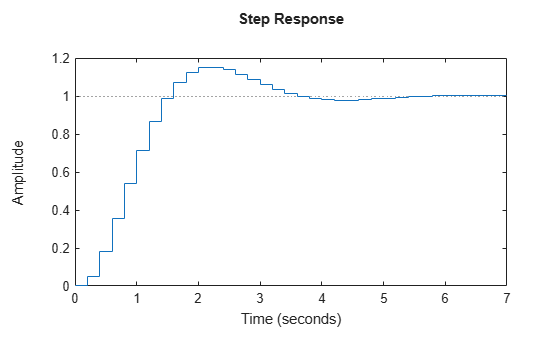The step response reflects the discretization of the model, showing the response computed every 0.2 seconds.

Examine the step response of the following transfer function.

sys = zpk(-1,[-0.2+3j,-0.2-3j],1) * tf([1 1],[1 0.05])
sys =

(s+1)^2
----------------------------
(s+0.05) (s^2 + 0.4s + 9.04)

Continuous-time zero/pole/gain model.
step(sys)By default, step chooses an end time that shows the steady state that the response is trending toward. This system has fast transients, however, which are obscured on this time scale. To get a closer look at the transient response, limit the step plot to t = 15 s.

step(sys,15)Alternatively, you can specify the exact times at which you want to examine the step response, provided they are separated by a constant interval. For instance, examine the response from the end of the transient until the system reaches steady state.

t = 20:0.2:120;
step(sys,t)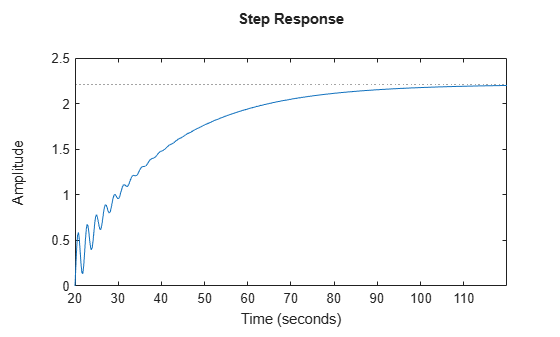Even though this plot begins at t = 20, step always applies the step input at t = 0.

Consider the following second-order state-space model:

$\begin{array}{l}\left[\begin{array}{c}{\underset{}{\overset{˙}{x}}}_{1}\\ {\underset{}{\overset{˙}{x}}}_{2}\end{array}\right]=\left[\begin{array}{cc}-0.5572& -0.7814\\ 0.7814& 0\end{array}\right]\left[\begin{array}{c}{x}_{1}\\ {x}_{2}\end{array}\right]+\left[\begin{array}{cc}1& -1\\ 0& 2\end{array}\right]\left[\begin{array}{c}{u}_{1}\\ {u}_{2}\end{array}\right]\\ y=\left[\begin{array}{cc}1.9691& 6.4493\end{array}\right]\left[\begin{array}{c}{x}_{1}\\ {x}_{2}\end{array}\right]\end{array}$

A = [-0.5572,-0.7814;0.7814,0];
B = [1,-1;0,2];
C = [1.9691,6.4493];
sys = ss(A,B,C,0);

This model has two inputs and one output, so it has two channels: from the first input to the output, and from the second input to the output. Each channel has its own step response.

When you use step, it computes the responses of all channels.

step(sys)The left plot shows the step response of the first input channel, and the right plot shows the step response of the second input channel. Whenever you use step to plot the responses of a MIMO model, it generates an array of plots representing all the I/O channels of the model. For instance, create a random state-space model with five states, three inputs, and two outputs, and plot its step response.

step(sys)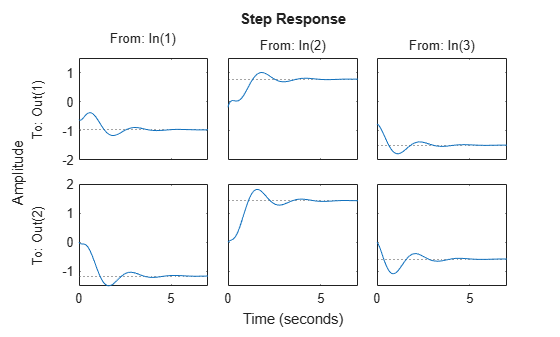In a MATLAB figure window, you can restrict the plot to a subset of channels by right-clicking on the plot and selecting I/O Selector.

step allows you to plot the responses of multiple dynamic systems on the same axis. For instance, compare the closed-loop response of a system with a PI controller and a PID controller. Create a transfer function of the system and tune the controllers.

H = tf(4,[1 2 10]);
C1 = pidtune(H,'PI');
C2 = pidtune(H,'PID');

Form the closed-loop systems and plot their step responses.

sys1 = feedback(H*C1,1);
sys2 = feedback(H*C2,1);
step(sys1,sys2)
legend('PI','PID','Location','SouthEast')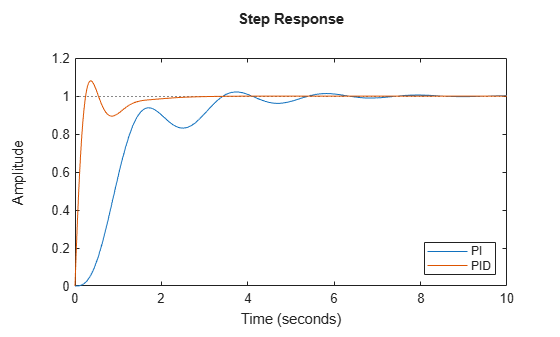By default, step chooses distinct colors for each system that you plot. You can specify colors and line styles using the LineSpec input argument.

step(sys1,'r--',sys2,'b')
legend('PI','PID','Location','SouthEast')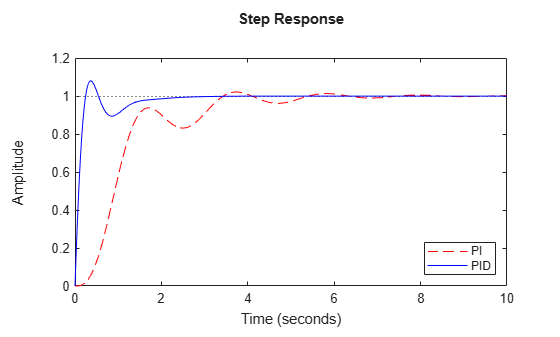The first LineSpec 'r--' specifies a dashed red line for the response with the PI controller. The second LineSpec 'b' specifies a solid blue line for the response with the PID controller. The legend reflects the specified colors and linestyles. For more plot customization options, use stepplot.

The example Compare Responses of Multiple Systems shows how to plot responses of several individual systems on a single axis. When you have multiple dynamic systems arranged in a model array, step plots all their responses at once.

Create a model array. For this example, use a one-dimensional array of second-order transfer functions having different natural frequencies. First, preallocate memory for the model array. The following command creates a 1-by-5 row of zero-gain SISO transfer functions. The first two dimensions represent the model outputs and inputs. The remaining dimensions are the array dimensions.

sys = tf(zeros(1,1,1,5));

Populate the array.

w0 = 1.5:1:5.5;    % natural frequencies
zeta = 0.5;        % damping constant
for i = 1:length(w0)
sys(:,:,1,i) = tf(w0(i)^2,[1 2*zeta*w0(i) w0(i)^2]);
end

(For more information about model arrays and how to create them, see Model Arrays (Control System Toolbox).) Plot the step responses of all models in the array.

step(sys)step uses the same linestyle for the responses of all entries in the array. One way to distinguish among entries is to use the SamplingGrid property of dynamic system models to associate each entry in the array with the corresponding w0 value.

sys.SamplingGrid = struct('frequency',w0);

Now, when you plot the responses in a MATLAB figure window, you can click a trace to see which frequency value it corresponds to.

When you give it an output argument, step returns an array of response data. For a SISO system, the response data is returned as a column vector of length equal to the number of time points at which the response is sampled. You can provide the vector t of time points, or allow step to select time points for you based on system dynamics. For instance, extract the step response of a SISO system at 101 time points between t = 0 and t = 5 s.

sys = tf(4,[1 2 10]);
t = 0:0.05:5;
y = step(sys,t);
size(y)
ans = 1×2

101     1

For a MIMO system, the response data is returned in an array of dimensions N-by-Ny-by-Nu, where Ny and Nu are the number of outputs and inputs of the dynamic system. For instance, consider the following state-space model, representing a two-input, one-output system.

A = [-0.5572,-0.7814;0.7814,0];
B = [1,-1;0,2];
C = [1.9691,6.4493];
sys = ss(A,B,C,0);

Extract the step response of this system at 200 time points between t = 0 and t = 20 s.

t = linspace(0,20,200);
y = step(sys,t);
size(y)
ans = 1×3

200     1     2

y(:,i,j) is a column vector containing the step response from the jth input to the ith output at the times t. For instance, extract the step response from the second input to the output.

y12 = y(:,1,2);
plot(t,y12)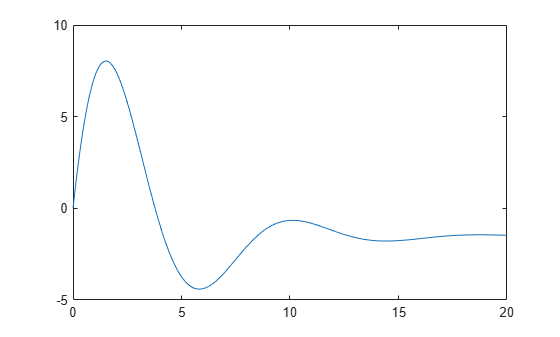Create a feedback loop with delay and plot its step response.

s = tf('s');
G = exp(-s) * (0.8*s^2+s+2)/(s^2+s);
sys = feedback(ss(G),1);
step(sys)The system step response displayed is chaotic. The step response of systems with internal delays may exhibit odd behavior, such as recurring jumps. Such behavior is a feature of the system and not software anomalies.

By default, step applies an input signal that changes from 0 to 1 at t = 0. To customize the amplitude and offset, use stepDataOptions. For instance, compute the response of a SISO state-space model to a signal that changes from 1 to –1 to at t = 0.

A = [1.6 -0.7;
1  0];
B = [0.5; 0];
C = [0.1 0.1];
D = 0;
sys = ss(A,B,C,D,0.2);

opt = stepDataOptions;
opt.InputOffset = 1;
opt.StepAmplitude = -2;

step(sys,opt)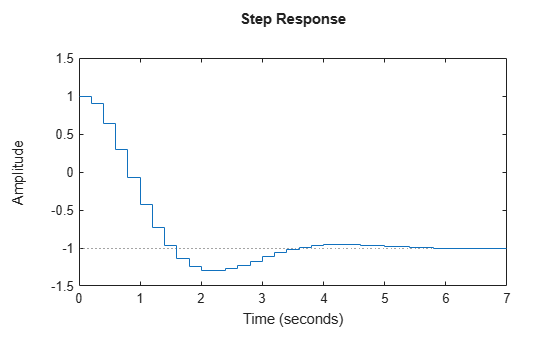For responses to arbitrary input signals, use lsim (Control System Toolbox).

Compare the step response of a parametric identified model to a non-parametric (empirical) model. Also view their 3 $\sigma$ confidence regions.

Estimate a parametric model.

sys1 = ssest(z1,4);

Estimate a non-parametric model.

sys2 = impulseest(z1);

Plot the step responses for comparison.

t = (0:0.1:10)';
[y1, ~, ~, ysd1] = step(sys1,t);
[y2, ~, ~, ysd2] = step(sys2,t);
plot(t, y1, 'b', t, y1+3*ysd1, 'b:', t, y1-3*ysd1, 'b:')
hold on
plot(t, y2, 'g', t, y2+3*ysd2, 'g:', t, y2-3*ysd2, 'g:')Compute the step response of an identified time-series model.

A time-series model, also called a signal model, is one without measured input signals. The step plot of this model uses its (unmeasured) noise channel as the input channel to which the step signal is applied.

Estimate a time-series model.

sys = ar(z9, 4);

ys is a model of the form A y(t) = e(t) , where e(t) represents the noise channel. For computation of step response, e(t) is treated as an input channel, and is named e@y1.

Plot the step response.

step(sys)Validate the linearization of a nonlinear ARX model by comparing the small amplitude step responses of the linear and nonlinear models.

Estimate a nonlinear ARX model.

nlsys = nlarx(z2,[4 3 10],idTreePartition,'custom',{'sin(y1(t-2)*u1(t))+y1(t-2)*u1(t)+u1(t).*u1(t-13)','y1(t-5)*y1(t-5)*y1(t-1)'},'nlr',[1:5, 7 9]);

Determine an equilibrium operating point for nlsys corresponding to a steady-state input value of 1.

u0 = 1;
y0 = r.SignalLevels.Output;

Obtain a linear approximation of nlsys at this operating point.

sys = linearize(nlsys,u0,X);

Validate the usefulness of sys by comparing its small-amplitude step response to that of nlsys.

The nonlinear system nlsys is operating at an equilibrium level dictated by (u0, y0). Introduce a step perturbation of size 0.1 about this steady-state and compute the corresponding response.

opt = stepDataOptions;
opt.InputOffset = u0;
opt.StepAmplitude = 0.1;
t = (0:0.1:10)';
ynl = step(nlsys, t, opt);

The linear system sys expresses the relationship between the perturbations in input to the corresponding perturbation in output. It is unaware of the nonlinear system's equilibrium values.

Plot the step response of the linear system.

opt = stepDataOptions;
opt.StepAmplitude = 0.1;
yl = step(sys, t, opt);

Add the steady-state offset, y0 , to the response of the linear system and plot the responses.

plot(t, ynl, t, yl+y0)
legend('Nonlinear', 'Linear with offset')Input Arguments

collapse all

Dynamic system, specified as a SISO or MIMO dynamic system model or array of dynamic system models. Dynamic systems that you can use include:

• Continuous-time or discrete-time numeric LTI models, such as tf, zpk, or ss models.

• Generalized or uncertain LTI models such as genss or uss models. (Using uncertain models requires Robust Control Toolbox™ software.)

• For tunable control design blocks, the function evaluates the model at its current value for both plotting and returning response data.

• For uncertain control design blocks, the function plots the nominal value and random samples of the model. When you use output arguments, the function returns response data for the nominal model only.

• Sparse state-space models such as sparss and mechss models.

• Identified LTI models, such as idtf, idss, or idproc models. For such models, the function can also plot confidence intervals and return standard deviations of the frequency response. See Step Responses of Identified Models with Confidence Regions.

step does not support frequency-response data models such as frd, genfrd, or idfrd models.

If sys is an array of models, the function plots the responses of all models in the array on the same axes. See Step Response of Systems in a Model Array.

End time for the step response, specified as a positive scalar value. step simulates the step response from t = 0 to t = tFinal.

• For continuous-time systems, the function determines the step size and number of points automatically from system dynamics. Express tFinal in the system time units, specified in the TimeUnit property of sys.

• For discrete-time systems, the function uses the sample time of sys as the step size. Express tFinal in the system time units, specified in the TimeUnit property of sys.

• For discrete-time systems with unspecified sample time (Ts = -1), step interprets tFinal as the number of sampling periods to simulate.

Time vector at which to compute the step response, specified as a vector of positive scalar values. Express t in the system time units, specified in the TimeUnit property of sys.

• For continuous-time models, specify t in the form Ti:dt:Tf. To obtain the response at each time step, the function uses dt as the sample time of a discrete approximation to the continuous system (see Algorithms).

• For discrete-time models, specify t in the form Ti:Ts:Tf, where Ts is the sample time of sys.

step always applies the step input at t = 0, regardless of Ti.

Line style, marker, and color, specified as a string or vector of one, two, or three characters. The characters can appear in any order. You do not need to specify all three characteristics (line style, marker, and color). For example, if you omit the line style and specify the marker, then the plot shows only the marker and no line. For more information about configuring this argument, see the LineSpec input argument of the plot function.

Example: 'r--' specifies a red dashed line

Example: '*b' specifies blue asterisk markers

Example: 'y' specifies a yellow line

Input offset and amplitude of the applied step signal, specified as a stepDataOptions option set. By default, step applies an input that goes from 0 to 1 at time t = 0. Use this input argument to change the initial and final values of the step input. See Response to Custom Step Input for an example.

Output Arguments

collapse all

Step response data, returned as an array.

• For SISO systems, y is a column vector of the same length as t (if provided) or tOut (if you do not provide t).

• For single-input, multi-output systems, y is a matrix with as many rows as there are time samples and as many columns as there are outputs. Thus, the jth column of y, or y(:,j), contains the step response of from the input to the jth output.

• For MIMO systems, the step responses of each input channel are stacked up along the third dimension of y. The dimensions of y are then N-by-Ny-by-Nu, where:

• N is the number of time samples.

• Ny is the number of system outputs.

• Nu is the number of system inputs.

Thus, y(:,i,j) is a column vector containing the step response from the jth input to the ith output at the times specified in t or tOut.

Times at which step response is computed, returned as a vector. When you do not provide a specific vector t of times, step chooses this time vector based on the system dynamics. The times are expressed in the time units of sys.

State trajectories, returned as an array. When sys is a state-space model, x contains the evolution of the states of sys at each time in t or tOut. The dimensions of x are N-by-Nx-by-Nu, where:

• N is the number of time samples.

• Nx is the number of states.

• Nu is the number of system inputs.

Thus, the evolution of the states in response to a step injected at the kth input is given by the array x(:,:,k). The row vector x(i,:,k) contains the state values at the ith time step.

Standard deviation of the step response of an identified model, returned as an array of the same dimensions as y. If sys does not contain parameter covariance information, then ysd is empty.

Tips

• To simulate system responses to arbitrary input signals, use lsim.

Algorithms

To obtain samples of continuous-time models without internal delays, step converts such models to state-space models and discretizes them using a zero-order hold on the inputs. step chooses the sampling time for this discretization automatically based on the system dynamics, except when you supply the input time vector t in the form t = 0:dt:Tf. In that case, step uses dt as the sampling time. The resulting simulation time steps tOut are equisampled with spacing dt.

For systems with internal delays, Control System Toolbox™ software uses variable step solvers. As a result, the time steps tOut are not equisampled.

 L.F. Shampine and P. Gahinet, "Delay-differential-algebraic equations in control theory," Applied Numerical Mathematics, Vol. 56, Issues 3–4, pp. 574–588.

System Identification Toolbox DocumentationGet trial now